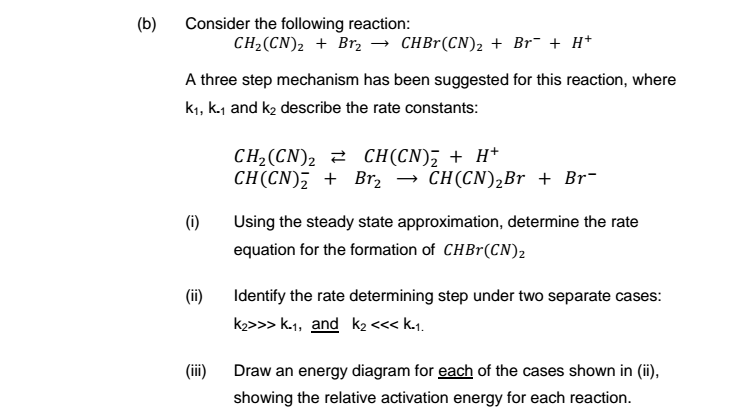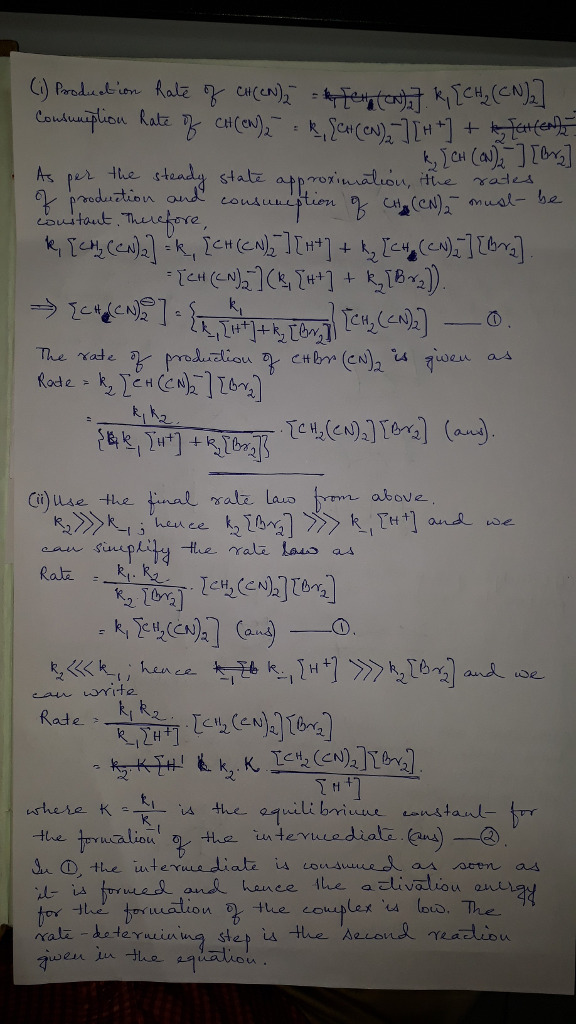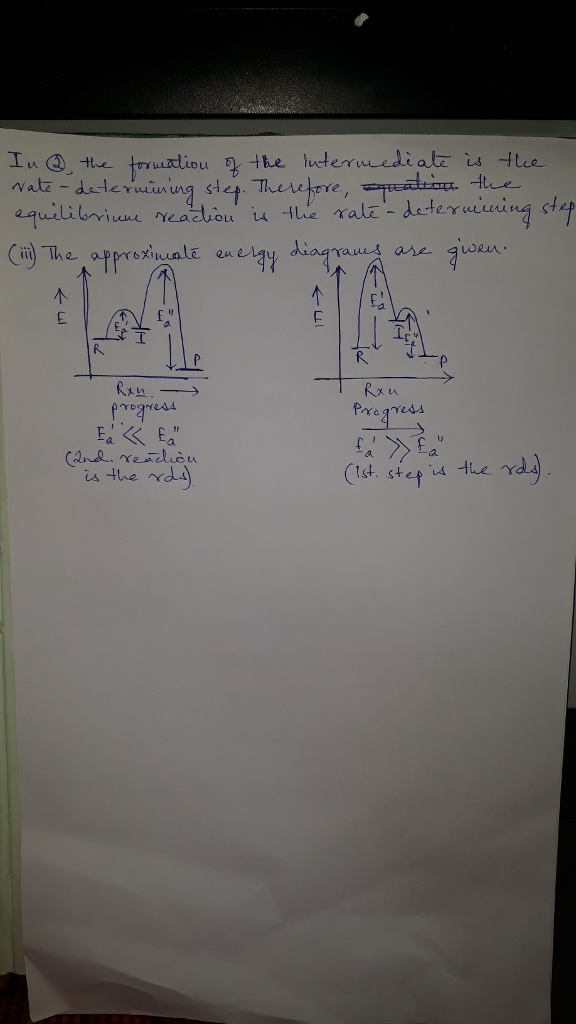# Question & Answer: Consider the following reaction: CH_2(CN)_2 + Br_2 rightarrow CHBr(CN)_2 + Br^- + H^+…..Consider the following reaction: CH_2(CN)_2 + Br_2 rightarrow CHBr(CN)_2 + Br^- + H^+ A three step mechanism has been suggested for this reaction, where k_1, k._1 and k_2 describe the rate constants: CH_2(CN)_2 CH(CN)^-_2 + H^+ CH(CN)^-_2 + Br_2 rightarrow CH(CN)_2Br + Br^- (i) Using the steady state approximation, determine the rate equation for the formation of CHBr(CN)_2 (ii) Identify the rate determining step under two separate cases: k_2 > > > k._1 and k_2